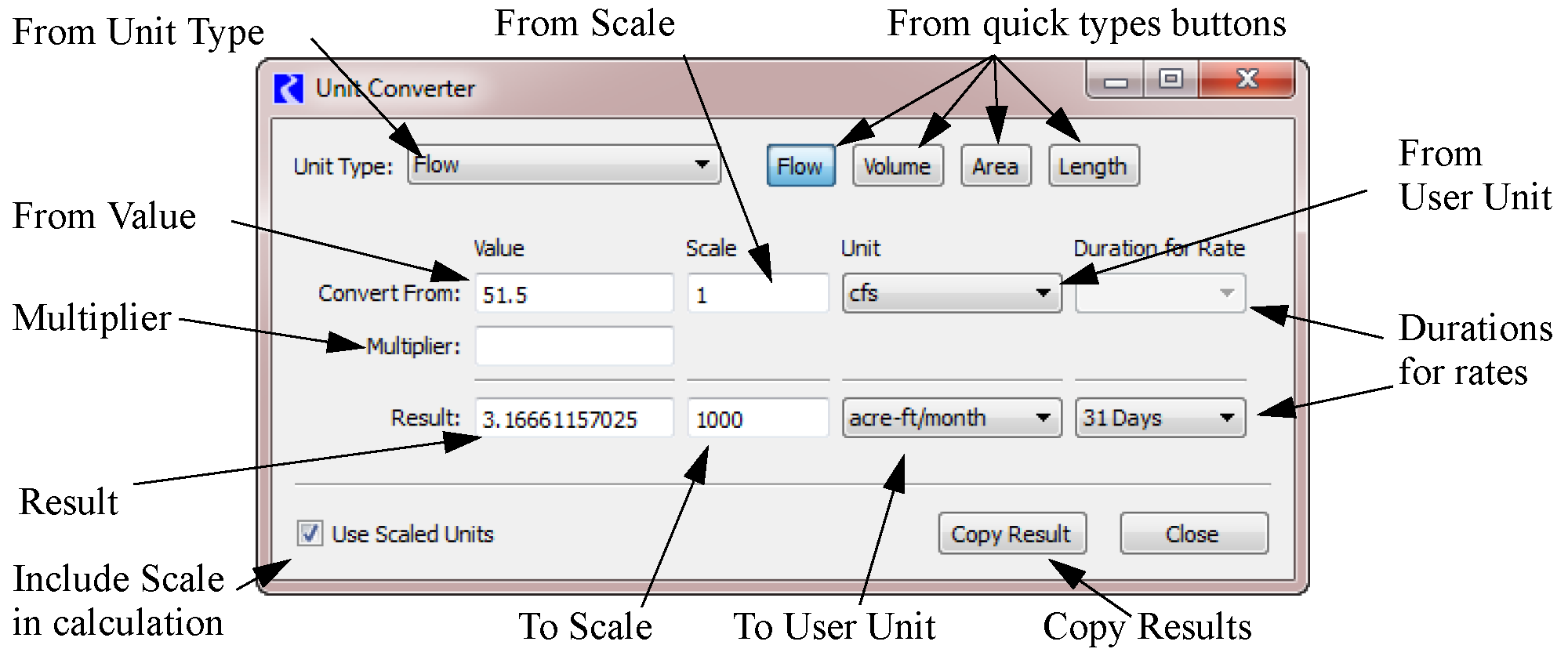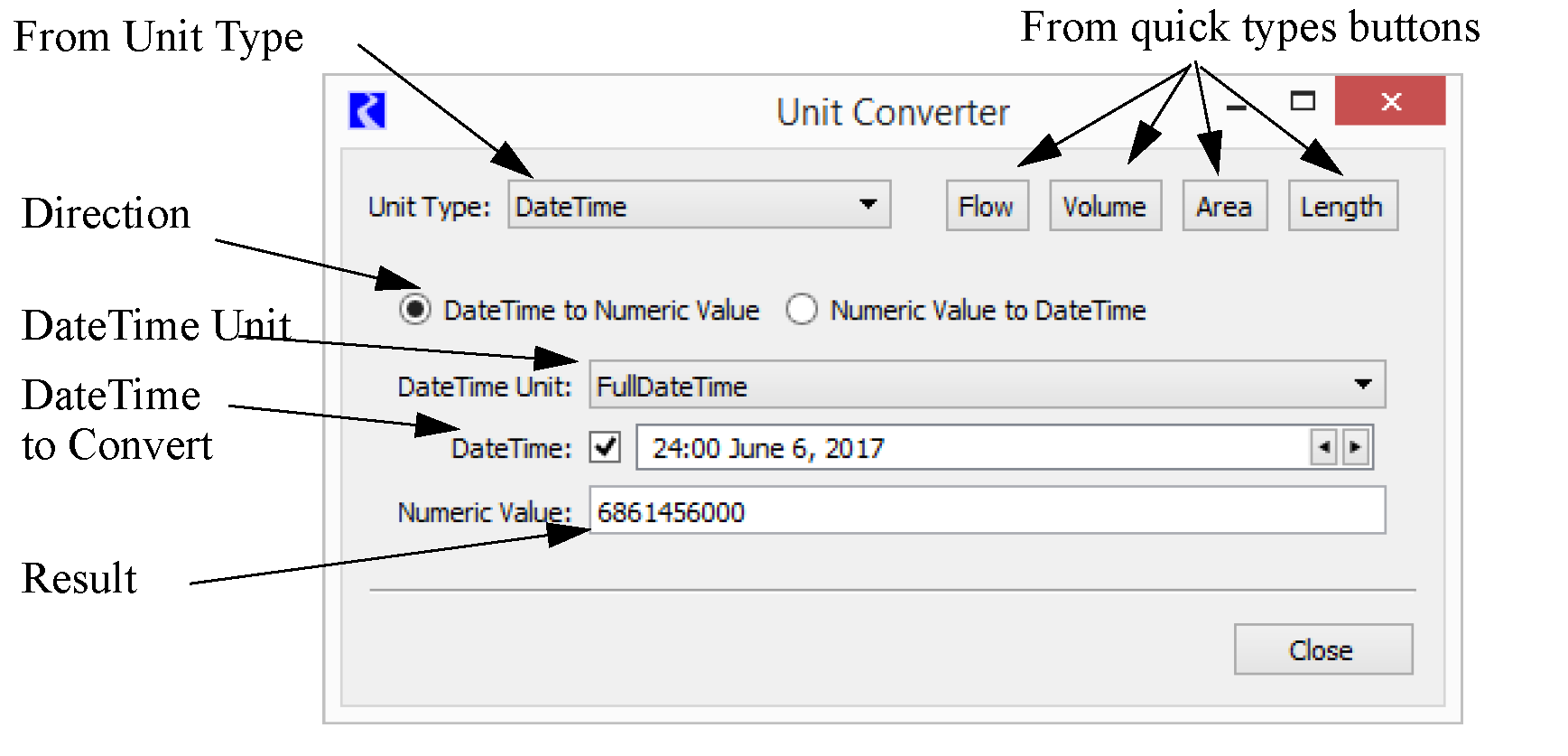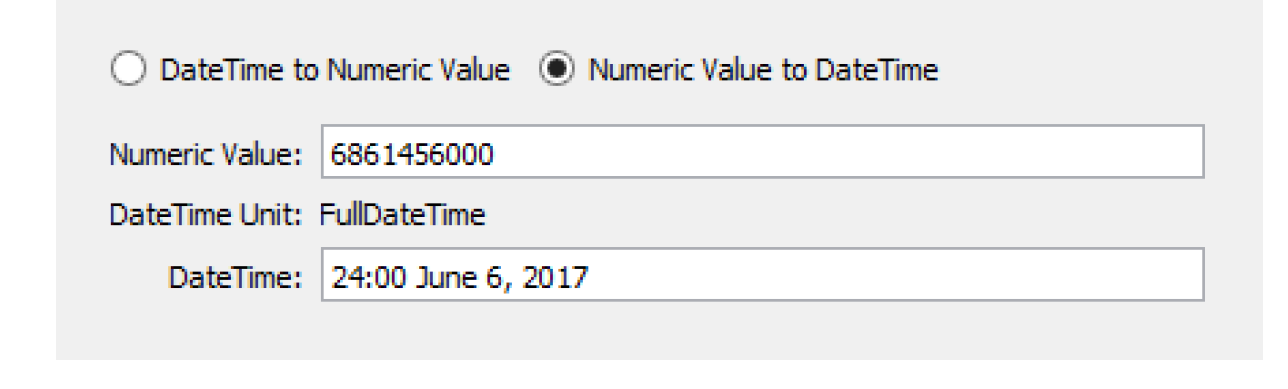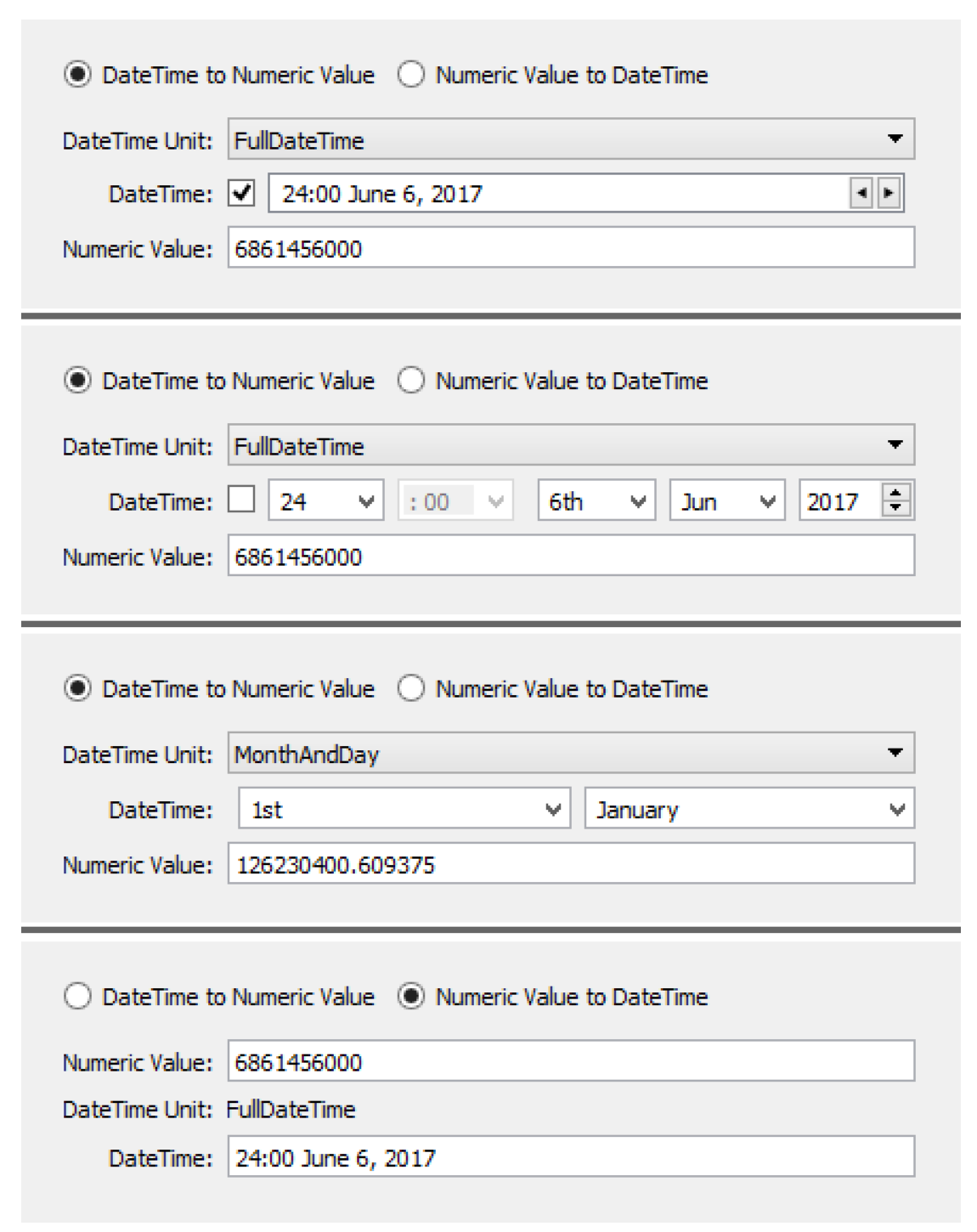User Interface : Units : Unit Converter Dialog
Unit Converter Dialog
The Unit Converter utility is a small calculator on the workspace which converts values using the same conversion factors used internally by RiverWare. To access the dialog, from the main workspace, select Units, then Unit Converter.
Converting Numeric Values
Figure 3.6 shows the Unit Converter dialog with all of the features displayed for numeric values.
Figure 3.6Following is the basic order in which the dialog is used and the options available.
1. Unit Type. Select the unit type for which the calculation should be done. Use the quick types buttons to select one of the four common types (Flow, Volume, Area, or Length) or use the pull-down menu to choose one of the less common types.
2. Value. Input a value to Convert From in the text box
3. Scale. Specify whether on not to use scale in either the from value or the result by toggling on the Include Scaled Units check box. When this box is checked, the Scale area is displayed. Then, enter a scale in the either of the two Scale text boxes.
4. Unit. Specify the user unit using the pull-down menu for both the from line and the result line. The unit menu contain all of the units of the selected type.
5. Duration for Rates. For units that include a rate (e.g. acre-feet/month) where the time scale is not constant (i.e. 1 Month or 1 Year), specify the duration. For monthly rates, select 28, 29, 30, or 31 days. For yearly rates, the specify 365 or 366 days.
6. Multiplier. Optionally enter a multiplier. The from value is converted to the units of the result and then multiplied by the Multiplier.
7. Result. The result is calculated immediately based on the specified input. The value which appears in the Result text field is now in terms of the new units, scale, and any specified multiplier.
8. Copy Result. You may wish to copy the result to the clipboard. Click the Copy Results button to copy the numerical value only. Hold down Shift and click Copy Result to copy the result and its scaled units.
The Unit Converter uses the conversion factors specified in the Units List dialog. To open the Units List dialog, from the Units menu in the main RiverWare workspace, select List Available Units. At the bottom left of the resulting Units List dialog, there is a check box to Show only units that are present on the workspace. Uncheck the box to show all available units and their conversion factors.
Converting DateTime Values to Numeric Values
Slots can store DateTime values; see DateTime Values in Slots for details. Internally, the dates are stored as numeric values. Sometimes, it is useful to convert the DateTime values shown to their encoded numbers. The Unit Converter provides one way to do this. Figure 3.7 shows the Unit Converter dialog with all the features displayed for DateTime values.
Figure 3.7When DateTime is the selected unit type, a different set of controls as shown.
Indicate the direction of the conversion by selecting one of the following options:
• DateTime to Numeric Value
• Numeric Value to DateTime
Following is the basic order in which the dialog is used and the options available.
1. In the DateTime to Numeric Value direction (shown in Figure 3.7), select the DateTime Unit (one of which is FullDateTime—fully specified absolute dates and times). The selected unit determines which controls are presented according to the relevant parts of a DateTime value. In the FullDateTime case, an additional checkbox switches between a conventional DateTime entry box to individual DateTime part controls.
2. When the Numeric Value to DateTime option is selected (shown in Figure 3.8), enter a numeric value (often you would copy/paste this as needed), and both the DateTime unit and value are displayed for that value.
Figure 3.8In both modes, the result is automatically computed as the inputs are modified. The result is selectable and copyable from the result field.
RiverWare supports the following units for the DateTime unit type:
• FullDateTime
• Month
• MonthAndDay
• TimeOfYear
• DayOfMonth
• TimeOfMonth
• TimeOfDay
• Year
Figure 3.9 shows units and controls.
Note:  DateTime values are stored in slots as floating point numbers. The integer part is the number of seconds since the beginning of 1800 (or, as RiverWare presents this, December 31, 1799, 24:00). For ordinary FullDateTimes, the fractional part is zero. Otherwise, the fractional part encodes which partial date time information is relevant. Year, Month, Day, Hour, Minutes, and Seconds.
Figure 3.9Revised: 01/04/2021